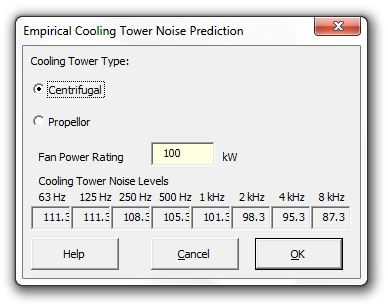### Strutt Help

Empirical Cooling Tower Noise Prediction    1/1

Strutt|Input Data|Empirical|Cooling Tower inserts sound power levels into the active row of the worksheet.Centrifugal calculates the overall sound power level using the formulae:
L_W = 85 + 11log_10(kW) (dB re 10-12 W); for fan power up to 60 kW

L_W = 93 + 7log_10(kW) (dB re 10-12 W); for fan power greater than 60 kW

Octave band sound power levels are then calculated by subtracting corrections from Table 11.7 (Bies and Hansen).

Propellor calculates the overall sound power level using the formulae:
L_W = 100 + 8log_10(kW) (dB re 10-12 W); for fan power up to 75 kW

L_W = 96 + 10log_10(kW) (dB re 10-12 W); for fan power greater than 75 kW

Octave band sound power levels are then calculated by subtracting corrections from Table 11.7 (Bies and Hansen).

References:

• Bies, D., Hansen, C., Engineering Noise Control, pp519 - 521